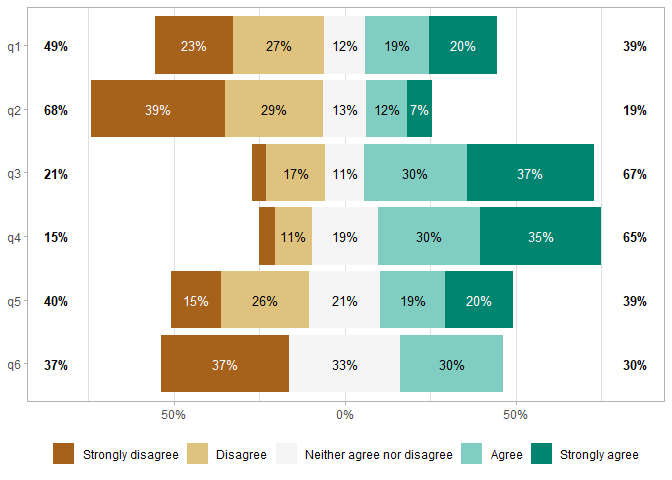The ggstats package provides suite of functions to plot regression model coefficients (“forest plots”) using ggplot2..

The suite also includes new statistics to compute proportions, weighted mean and cross-tabulation statistics, as well as new geometries to add alternative background color to a plot.

The original versions of several functions were originally developed within the GGally package.

## Installation & Documentation

To install stable version:

install.packages("ggstats")

Documentation of stable version: https://larmarange.github.io/ggstats/

To install development version:

remotes::install_github("larmarange/ggstats")

Documentation of development version: https://larmarange.github.io/ggstats/dev/

## Plot model coefficients

library(ggstats)

mod1 <- lm(Fertility ~ ., data = swiss)
ggcoef_model(mod1)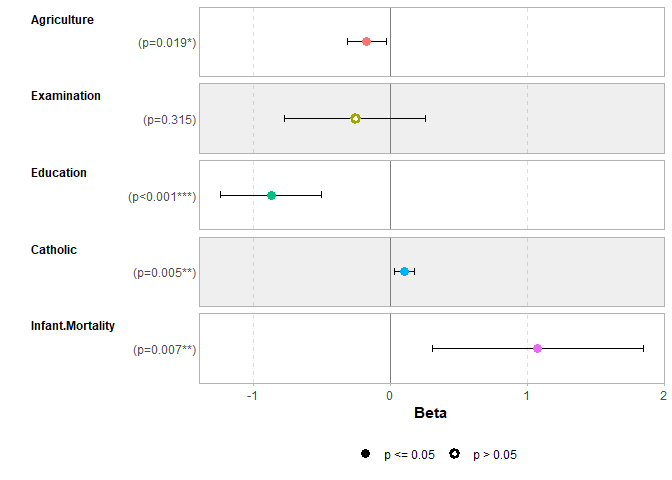## Comparing several models

mod2 <- step(mod1, trace = 0)
mod3 <- lm(Fertility ~ Agriculture + Education * Catholic, data = swiss)
models <- list(
"Full model" = mod1,
"Simplified model" = mod2,
"With interaction" = mod3
)

ggcoef_compare(models, type = "faceted")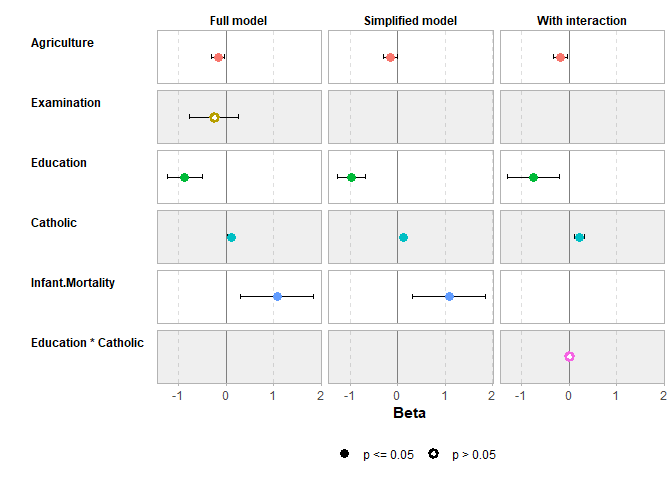## Compute custom proportions

library(ggplot2)
ggplot(as.data.frame(Titanic)) +
aes(x = Class, fill = Survived, weight = Freq, by = Class) +
geom_bar(position = "fill") +
geom_text(stat = "prop", position = position_fill(.5)) +
facet_grid(~ Sex)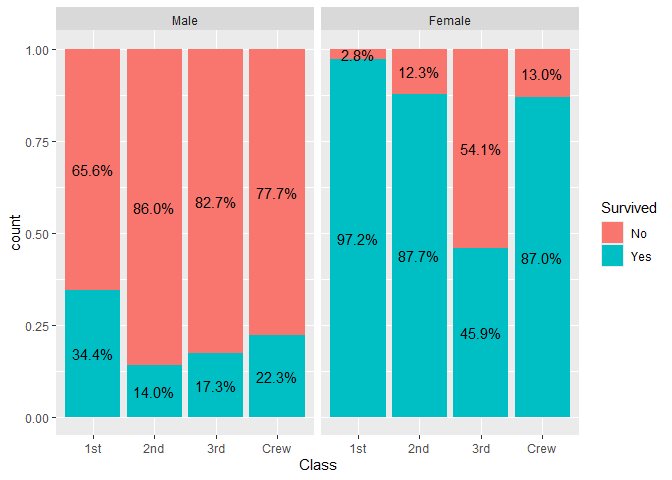## Compute weighted mean

data(tips, package = "reshape")
ggplot(tips) +
aes(x = day, y = total_bill, fill = sex) +
stat_weighted_mean(geom = "bar", position = "dodge") +
ylab("Mean total bill per day and sex")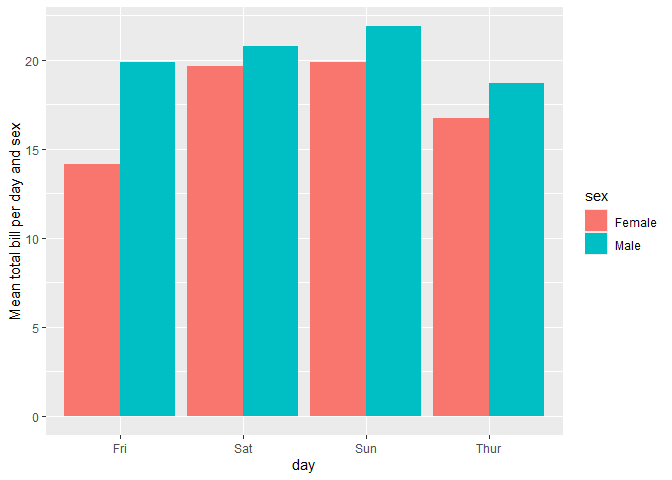## Compute cross-tabulation statistics

ggplot(as.data.frame(Titanic)) +
aes(
x = Class, y = Survived, weight = Freq,
size = after_stat(observed), fill = after_stat(std.resid)
) +
stat_cross(shape = 22) +
scale_fill_steps2(breaks = c(-3, -2, 2, 3), show.limits = TRUE) +
scale_size_area(max_size = 20)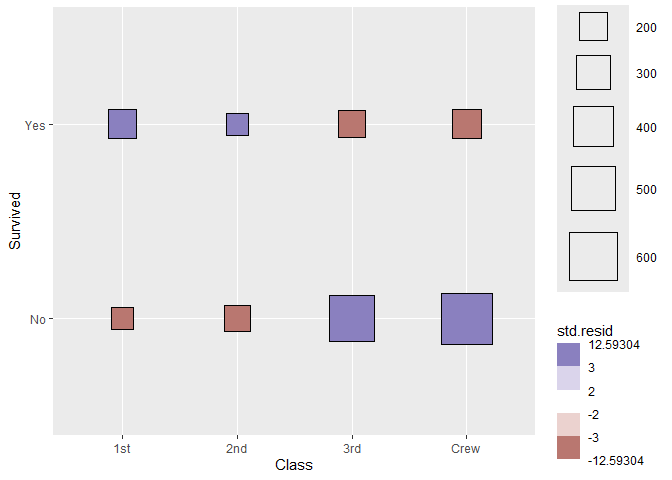## Plot survey objects taking into account weights

library(survey, quietly = TRUE)
#>
#> Attachement du package : 'survey'
#> L'objet suivant est masqué depuis 'package:graphics':
#>
#>     dotchart
dw <- svydesign(
ids = ~ 1,
weights = ~ Freq,
data = as.data.frame(Titanic)
)
ggsurvey(dw) +
aes(x = Class, fill = Survived) +
geom_bar(position = "fill") +
ylab("Weighted proportion of survivors")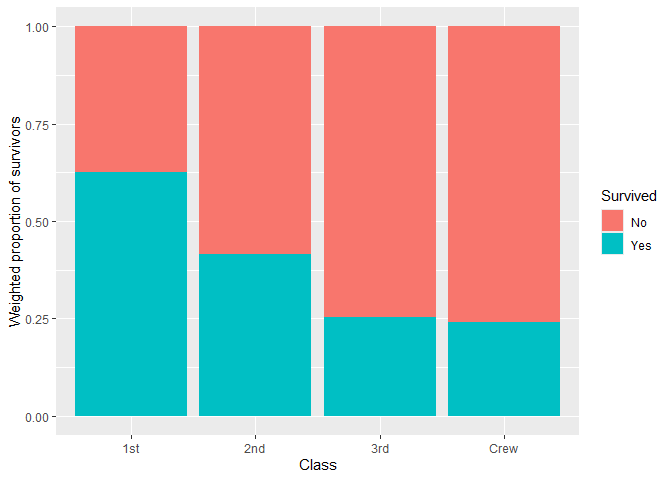## Plot Likert-type items

library(dplyr)
#>
#> Attachement du package : 'dplyr'
#> Les objets suivants sont masqués depuis 'package:stats':
#>
#>     filter, lag
#> Les objets suivants sont masqués depuis 'package:base':
#>
#>     intersect, setdiff, setequal, union
likert_levels <- c(
"Strongly disagree",
"Disagree",
"Neither agree nor disagree",
"Agree",
"Strongly agree"
)
set.seed(42)
df <-
tibble(
q1 = sample(likert_levels, 150, replace = TRUE),
q2 = sample(likert_levels, 150, replace = TRUE, prob = 5:1),
q3 = sample(likert_levels, 150, replace = TRUE, prob = 1:5),
q4 = sample(likert_levels, 150, replace = TRUE, prob = 1:5),
q5 = sample(c(likert_levels, NA), 150, replace = TRUE),
q6 = sample(likert_levels, 150, replace = TRUE, prob = c(1, 0, 1, 1, 0))
) %>%
mutate(across(everything(), ~ factor(.x, levels = likert_levels)))

gglikert(df)